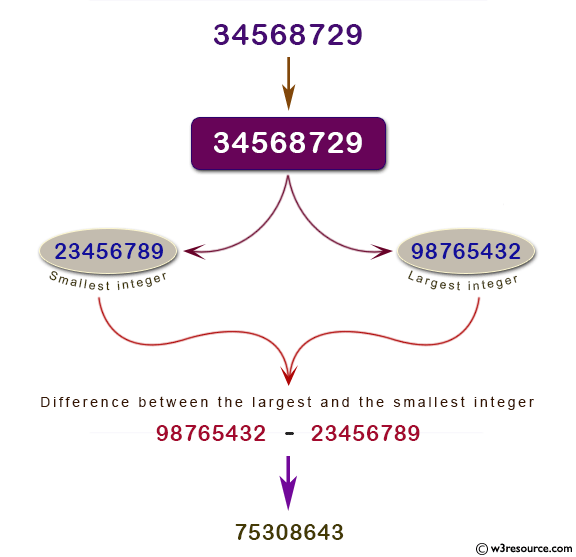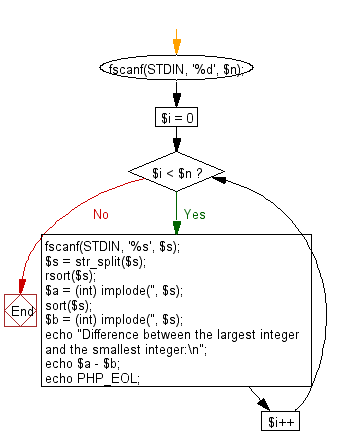﻿ PHP Exercise: Find the difference between the largest integer and the smallest integer - w3resource

# PHP Exercises: Find the difference between the largest integer and the smallest integer

## PHP: Exercise-64 with Solution

Write a PHP program to find the difference between the largest integer and the smallest integer which are created by 8 numbers from 0 to 9. The number that can be rearranged shall start with 0 as in 00135668.

Input: The difference between the largest integer and the smallest integer.

Pictorial Presentation:Sample Solution: -

PHP Code:

``````<?php
fscanf(STDIN, '%d', \$n);
for (\$i = 0; \$i < \$n; \$i++) {
fscanf(STDIN, '%s', \$s);
\$s = str_split(\$s);
rsort(\$s);
\$a = (int) implode('', \$s);
sort(\$s);
\$b = (int) implode('', \$s);
echo "Difference between the largest integer and the smallest integer:\n";
echo \$a - \$b;
echo PHP_EOL;
}
?>
```
```

Sample Input:
1
34568729

Sample Output:

```Difference between the largest integer and the smallest integer:
75308643```

Flowchart:PHP Code Editor:

Have another way to solve this solution? Contribute your code (and comments) through Disqus.

What is the difficulty level of this exercise?

Test your Programming skills with w3resource's quiz.

﻿

## PHP: Tips of the Day

Returns all elements in an array except for the first one

Example:

```<?php
function tips_tail(\$items)
{
return count(\$items) > 1 ? array_slice(\$items, 1) : \$items;
}

print_r(tips_tail([1, 5, 7]));
?>
```

Output:

```Array
(
 => 5
 => 7
)
```